# Daily Kakuro

##How to playThis is an example of a completed Kakuro grid. The initial starting grid is completely empty, apart from the clues you are given. Your aim is to fill the grid so that the numbers in each row and column add up to the total given by the clue.
In this example, you can see that we have a highlighted clue of 11, so the three highlighted cells under it must add to 11, and 2 + 1 + 8 = 11 ✔. We also have a highlighted horizontal clue of 23, so the three highlighted cells to the right of that clue must add to 23, and 9 + 6 + 8 = 23 ✔.
Duplicate numbers in each 'run' of cells is not allowed. Looking again at the clue and run of three cells we have just seen, you can see that the numbers 6, 8, and 9 are used again in the same row, but since they are in a different 'run', this is not against the rules.This is an example of a Kakuro starting grid. The first clues to look at are those where there is only 2 cells, and only one way to make that number. These are the totals we are interested in for clues with 2 cells:
• 3 (1+2)
• 4 (1+3)
• 16 (7+9)
• 17 (8+9)
I have highlighted all of the instances of this in the puzzle.
The cells we are particularly interested in are those where we have an intersection between these low/high numbers. Cell 'A' is one such example. There are two constraints on cell A,
• Looking at the vertical clue (3), it must be 1 or 2.
• Looking at the horizontal clue (4), it must be 1 or 3.
Combining these two constraints, and we can fill in cell A with a 1. The same logic can be applied for cell B.
It can also sometimes be useful to look for clues that have 3 cells with a low/high total. There is a horizontal clue of 6 in the bottom-left that only has 3 cells. There is only way to make 6 with 3 cells (1+2+3), so that can be useful sometimes.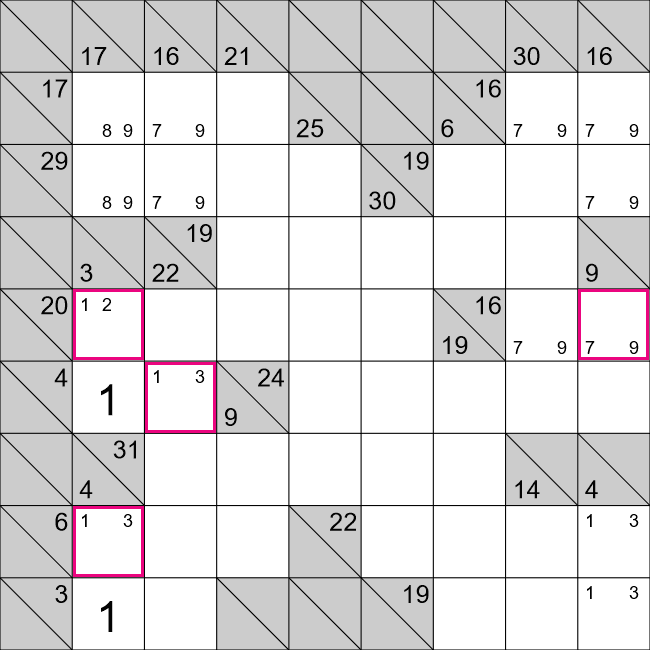We are now at this position. The three highlighted cells on the left side of this grid can also now be filled in - there is only one possible number for each.
We can now also look at the highlighted cell on the right side of this Kakuro puzzle. We already know from the horizontal clue (16) that this cell must be a 7 or a 9. This cell is also in a vertical clue of 9, which means that this cell must be 7; if this cell was 9 then that would mean the cell under it would have to be 0 to give a vertical total of 9, and 0 is not allowed.
We can also fill in the cell to it's left with a 9, and the cell under it with a 2.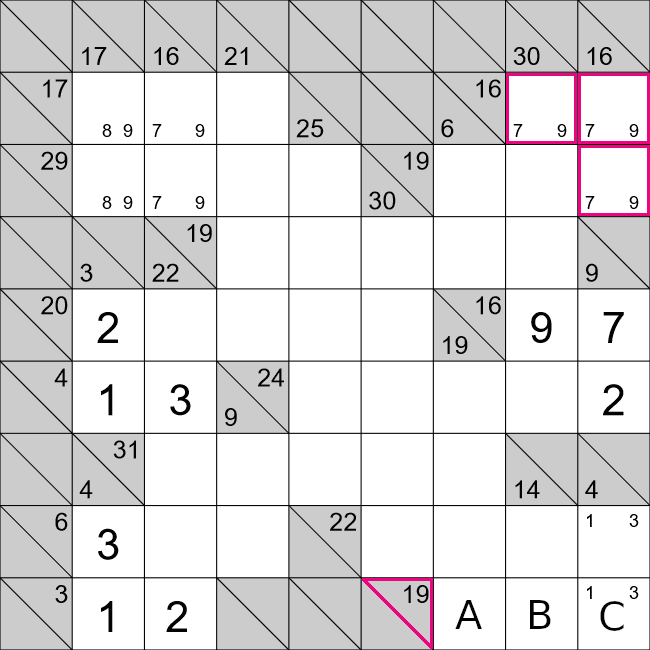We can now fill in the highlighted cells in the top right - look first at the vertical clue of 30, there is already a value of 9 in this column, so the top-most cell must be a 7.
We can also look at cells A, B, and C in the bottom-right. We already know that cell C must be 1 or 3. If cell C had a value of 1, then that would mean that cells A and B must add to 18, i.e. 9+9. It's not allowed to have the same number twice in the same 'run' of cells, so cell C must be 3.
Cells A and B must then add to 16, this is only possible with 9+7, so we can fill in some pencil marks for these cells as well.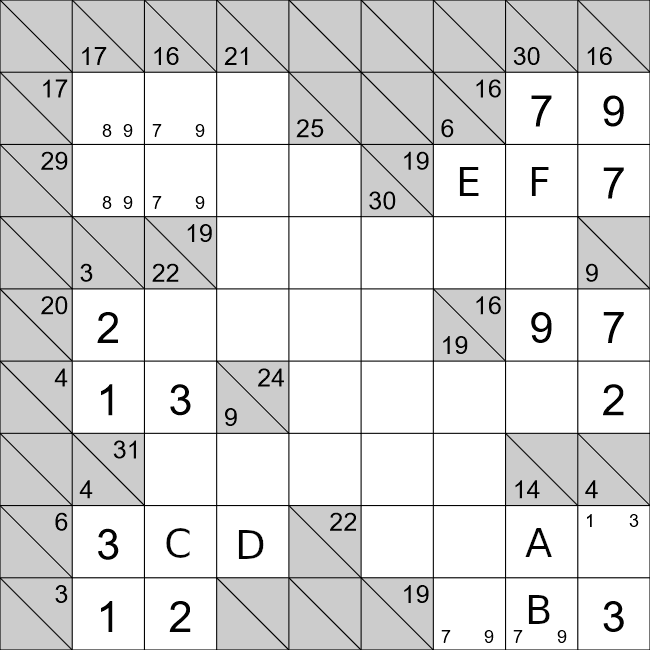We are now at this position. Cells A and B must add 14, with the pencil marks already in cell B, there are only two possibilities,
1. 14 = 7 + 7
2. 14 = 9 + 5
It's not allowed to have two sevens in the same run of cells like this, so the second combination must be the answer. Cell A must be 5, and cell B must be 9. This also allows us to fill in the cell to the left of B.
We can also fill in cells C and D. The only way to make 6 with three cells is 1+2+3. We already have a 3, there is already a 2 in the same vertical run as C, so that means that cell C must be a 1.
We can also enter some pencil marks for cells E and F. From the horizontal clue we have that 7 + E + F = 19, i.e. E + F = 12. Let's fill in those pencil marks for E and F, bearing in mind that there is already a 7 in that column of cells.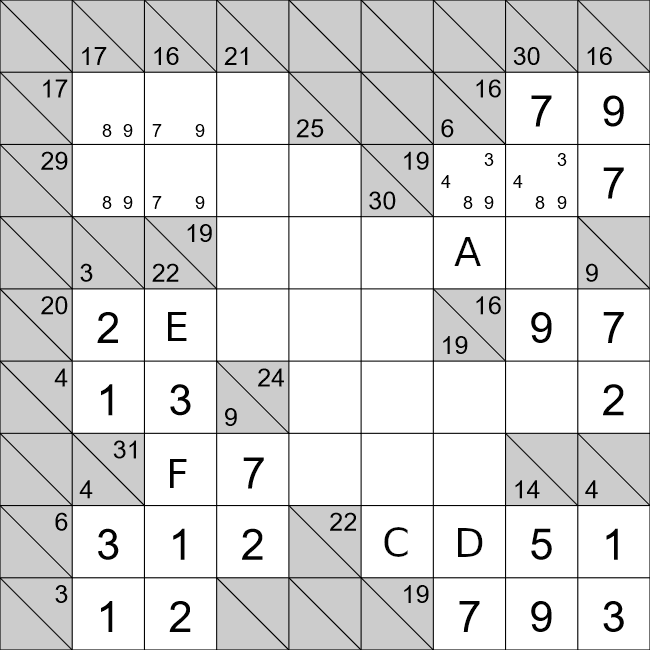We will first look at cell A. We know that cell A and the cell above it must add to 6. We already have some pencil marks for the cell above but we can remove all but one of them:
• 3: This would mean that cell A would also have to be 3, this isn't allowed.
• 4: This would mean cell A would be 2, this is OK.
• 8: This would mean cell A would be -2, this isn't allowed.
• 9: This would mean cell A would be -3, this isn't allowed.
The only valid value we have left for the cell above A is 4, and cell A must be 2!
Let's now look at cells C and D. We have that C + D + 5 + 1 = 22, i.e. C + D = 16. We already know that 16 with two cells is only possible one way, i.e. 16 = 7 + 9. There is already a 7 in the same vertical run of cells as D, so D must be a 9.
We can also look at cells E and F. We know that E + 3 + F + 1 + 2 = 22, i.e. E + F = 16. This means cells E and F can only be a 7 or 9. Cell F already has a 7 in this horizontal run of cells, so cell F must be a 9.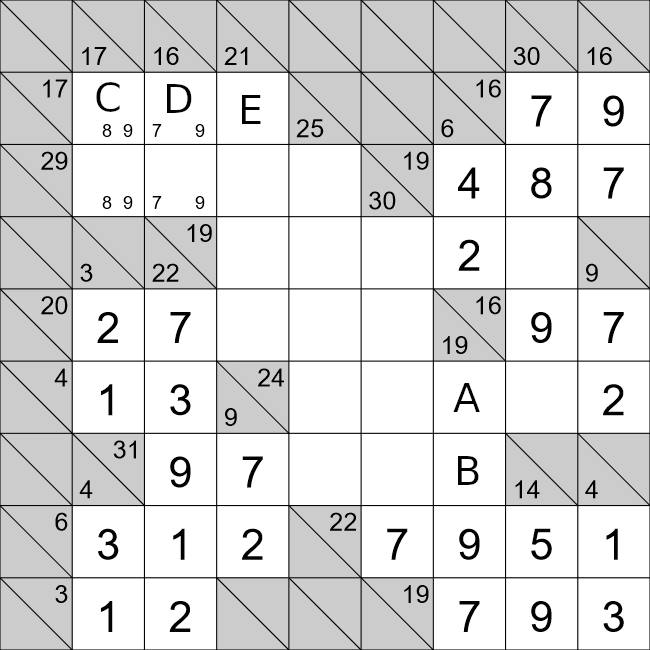We will now look at cells A and B. From looking at the vertical clue we have that 19 = A + B + 9 + 7, i.e. 3 = A + B. This means that both A and B must be 1 or 2. Cell A already has a 2 in that horizontal run, so cell A must be 1, and cell B must be 2.
We can also look at cells C, D and E. We already know that cell D must be 7 or 9. If cell D was 9, the smallest value C can take is 8, which already gives us a total of 8 + 9 = 17 for that horizontal run, i.e. Cell E would have to be 0, which is not allowed. This means that cell D must be 7, and we can fill in values for this whole block.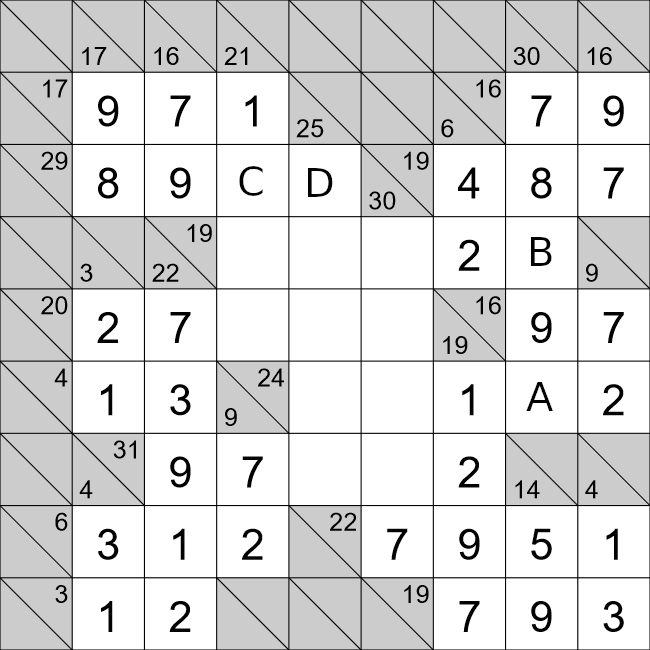We will now look at cells A and B. Looking horizontally, we already have,

30 = 7 + 8 + B + 9 + A
6 = B + A

There are a few different ways we can do this,
• 6 = 3 + 3. Can't have the same number twice in the same run of cells.
• 6 = 2 + 4. We already have a 2 in the same horizontal run as both A and B.
• 6 = 1 + 5. Only possibility, but only if cell B is 1, and cell A is 5.
We will also fill in the pencil marks for cells C and D. We have that 29 = 8 + 9 + C + D, i.e. 12 = C + D. There are a few ways we can make 12 with two cells:
• 12 = 9 + 3 ❌
• 12 = 8 + 4 ❌
• 12 = 7 + 5 ✔
The first two options aren't valid as there is already a 8 and 9 in that horizontal run of cells.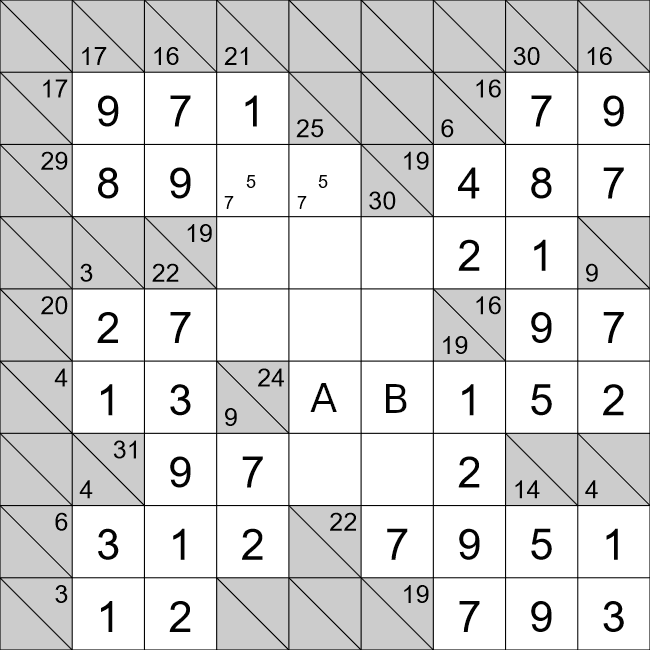We will now look at cells A and B. For this horizontal row we have that,

24=A+B+1+5+2
16=A+B.

There is only one way to make 16 with two cells, i.e. 16=7+9. Cell B already has a 7 in that vertical run of cells, so cell B must be 9, and cell A must be 7.
This also means that we can fill in the two cells at the top of the puzzle with the pencil marks of 5 and 7.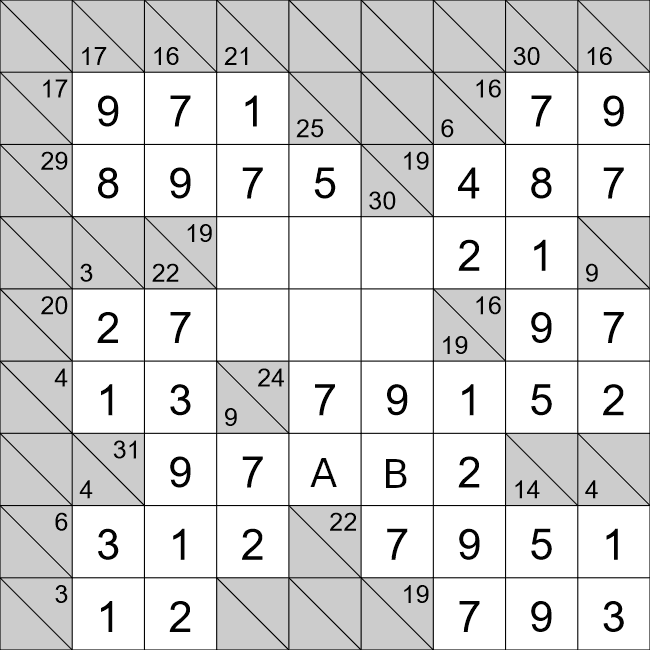We will now look at cells A and B. For this horizontal row we have,

31=9+7+A+B+2
13=A+B.

There are a few ways we can achieve this,
• 13 = 9 + 4 ❌
• 13 = 8 + 5 ✔
• 13 = 7 + 6 ❌
The first and third options are possible because we already have a 9 and 7 in that horizontal run of cells. That only leaves the second option. Cell A already has a 5 in that vertical run of cells, so cell A must 8, and cell B must be 5.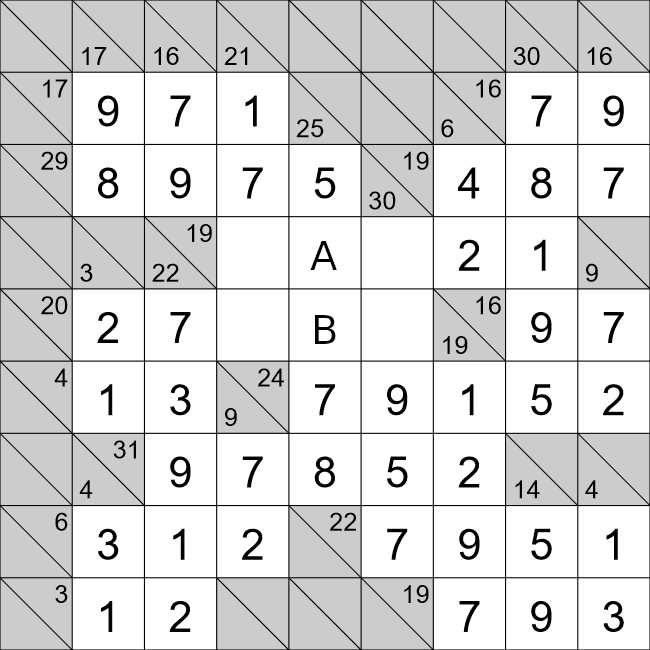We will now look at cells A and B. For this vertical row we have,

25=5+A+B+7+8
5=A+B.

There are a couple of different ways we can achieve this,
• 5 = 1 + 4 ✔
• 5 = 2 + 3 ❌
Cell A already has a 1 in that horizontal run, so cell A must be 4, and cell B must be 1.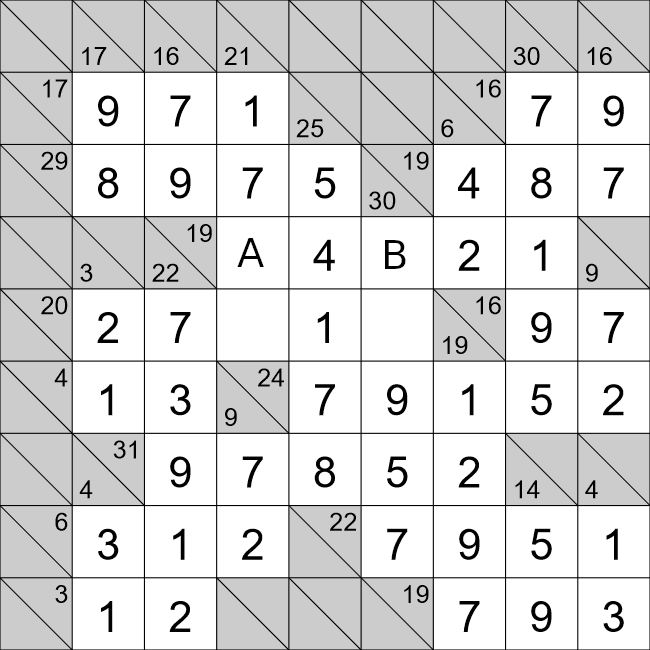We will now look at cells A and B. For this horizontal row we have,

19=A+4+B+2+1
12=A+B

Let's have a look at we can do this,
• 12 = 3 + 9 ✔
• 12 = 4 + 8 ❌ There is already a 4 in this row.
• 12 = 5 + 7 ❌ Cell B has a 5 and 7 in the same column.
As cell B already has a 9 in the same vertical run of cells, cell B must 3, and cell A must be 9.
We can also fill in the last two remaining cells to finish this Kakuro puzzle!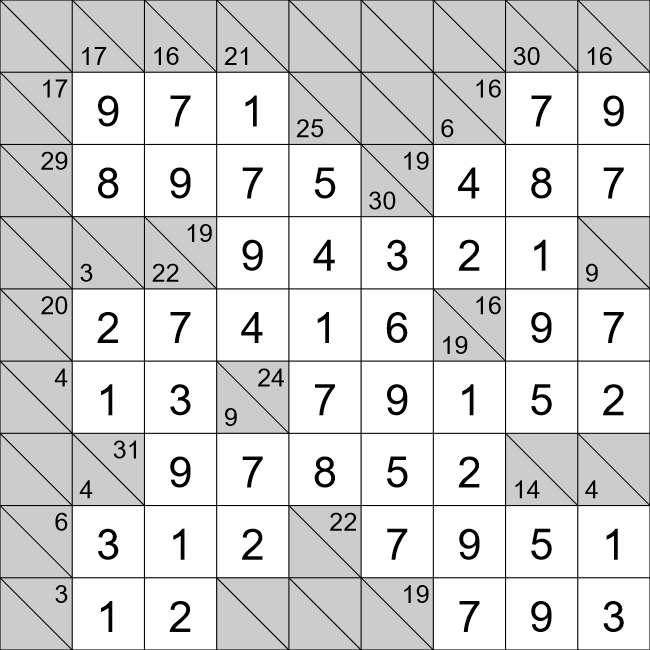We have finished this Kakuro puzzle!
The key to solving Kakuro puzzles is to look for pairs of cells that don't have many combinations.
At the beginning of the puzzle that means looking for cell pairs that add to 3, 4, 16, or 17.
As the puzzle progresses this will mean looking at rows and columns that are partially filled, but don't have many combinations available because of the cells already filled.